#Quadratic Equations: Completing the SquareFirst recall the algebraic identities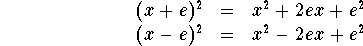We shall use these identities to carry out the process called Completing the Square. For example, consider the quadratic function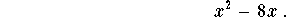What can be added to yield a perfect square? Using the previous identities, we see that if we put 2e=8, that is e=4, it is enough to add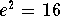to generate a perfect square. Indeed we have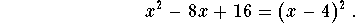It is not hard to generalize this to any quadratic function of the form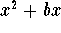. In this case, we have 2e=b which yields e=b/2. Hence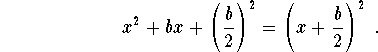Example: Use Complete the Square Method to solveSolution.First note that the previous ideas were developed for quadratic functions with no coefficient in front of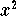. Therefore, let divide the equation by 2, to get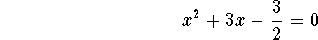which equivalent to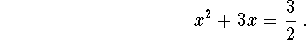In order to generate a perfect square we add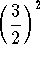to both sides of the equation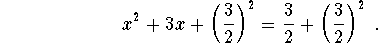Easy algebraic calculations give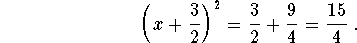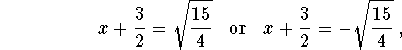which give the solutions to the equation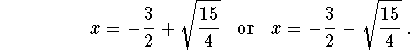We have developed a step-by-step procedure for solving a quadratic equation; or, in other words, an algorithm for solving a quadratic equation. This algorithm can be stated as a formula called Quadratic Formula.[Algebra] [Complex Variables]
[Geometry] [Trigonometry ]
[Calculus] [Differential Equations] [Matrix Algebra]S.O.S MATHematics home page

Do you need more help? Please post your question on our S.O.S. Mathematics CyberBoard.Author: Mohamed Amine Khamsi